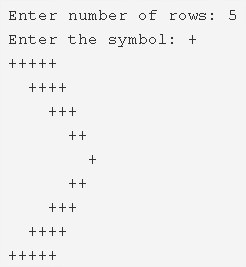# Right Arrow Star Pattern Program In C | 4 Ways

C program to print the right arrow star pattern – In this article, we will brief in on the several methods to print a right arrow star pattern in C programming.

Suitable examples and sample programs have also been added so that you can understand the whole thing very clearly. The compiler has also been added with which you can execute it yourself.

The ways used in this piece are as follows:

• Using For Loop
• Using While Loop
• Using Do-While Loop

As the name of the heading suggests, you need to print a right arrow star pattern.As it is suggested by the photo uploaded, firstly, you need to enter the number of rows. Then, the arrow will be printed accordingly.

Thus, the various ways to do so are as follows:

## Using For Loop

1. Read and store the entered rows number, entered a symbol into the variables n, ch.

2) The 1st for loop with the structure  for(i=0;i<n;i++) iterates through rows.

a) The 2nd for loop with the structure for(j=0;j<n;j++) iterates through columns.

If j<i this loop prints space. Otherwise, it prints symbol.

b) Cursor comes to next line for each iteration of i.

After above all iterations the half part of the left arrow star pattern will be printed.

3) The 3rd for loop iterates through rows with the structure for(i=2;i<=n;i++).

a) The 4th for loop iterates through columns with the structure for(j=0;j<n;j++).

If j<n-i then this loop prints space. Otherwise, it prints symbol.

b) Cursor comes to next line for each iteration of i.

After all iterations of the 4th loop, the remaining half part of the right arrow star pattern will be printed.

Output:

### Using While Loop

1. The while checks the condition then only executed the code if the condition is true. i=0, The while loop with the condition i<n iterates through rows

2) i=0, The 1st while loop with the condition i<n iterates through rows until the condition becomes false.

a) j=0, The 2nd while loop with the condition j<n iterates through columns until the condition becomes false.

If j<i, the loop prints space. Otherwise, it prints symbol.

j value increased by 1.

b) Cursor comes to next line.i value increased by 1.

After the above, while loops iterations the half part of the right arrow star pattern will be printed.

3) i=2, The 3rd while loop with the condition i<=n iterates through rows until the condition becomes false.

a) j=0, the while loop with the condition j<n iterates through columns until the condition becomes false.

If j<n-i this loop prints space, otherwise it prints symbol.

b) Cursor comes to next line.i value increased by 1.

After above two while loop iterations the remaining half part of the right arrow star pattern will be printed.

Output:

#### Using Do-While Loop

1. i=0, the 1st Do-while loop iterates through rows.

a) j=0, the 2nd do-while loop iterates through columns.

prints space if j<i, otherwise it prints symbol.

j value increased by 1.

Checks the condition j<n. If the condition is true then the 2nd loop iterates again.Repeats until the condition becomes false.

b) Cursor comes to next line.i value increased by 1.Checks the condition of 1st do-while loop i<n. If the condition is true then loop iterates again.Repeats until the condition becomes false.

2) i=2, The 3rd do-while loop iterates through rows.

a) j=0, the 4th do-while loop iterates through columns.

It prints space if j<n-i, otherwise it prints symbol.

j value increased by 1.Checks the condition j<n.

Checks the condition j<n. If the condition is true then the loop iterates again.Repeats until the condition becomes false.

b) Cursor comes to next line.i value increased by 1.Checks the condition i<=n. If the condition is true then the 3rd loop iterates again.Repeats until the condition becomes false.

Output:
x

## C Program To Print Number Of Days In A Month | Java Tutoring

C program to input the month number and print the number of days in that ...